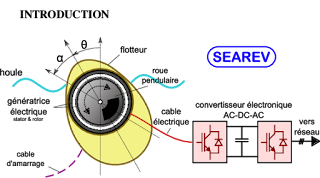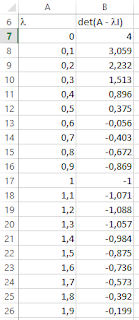## Articles

Affichage des articles du 2019

### Mine ventilation case calculationLet consider the following ventilation network in a mine. The network is made of five branches and four nodes. The airflow which gets in the mine is 47 m 3 /s. The Hardy Cross method will be used to compute the natural distribution of air flow in the different parts of the mine. Branch R Q 2 0.4 30 3 0.6 17 4 0.1 3 5 0.5 27 6 0.7 20 R is the airway resistance in kg/m 7 1st case We will start by considering an arbitrary distribution which respects the nodes law (the sum of incoming flows is equal to the sum of outgoing flows in a node) If the distribution is real, the sum of head losses along any loop is zero. The number of independent loops is given by b-n+1 where b is the number of branches and n the number of nodes. In the present case the number of loops is 5-4+1=2 The chosen loops are 243 and  564. We walk along the loops clockwise. If the f

### Répartition des débits d’air dans une mineSoit la mine suivante constituée de cinq branches et quatre noeuds Le débit qui entre dans la mine est de 47 m 3 /s. Nous allons utiliser la méthode de Hardy Cross pour trouver la répartition des débits dans les différents tronçons. Branche R Q 2 0.4 30 3 0.6 17 4 0.1 3 5 0.5 27 6 0.7 20 R est la résistance aéraulique en kg/m 7 1er cas On commence par une distribution arbitraire qui respecte la loi des nœuds (somme des débits arrivant au nœud est égal à la somme sortant du nœud) Si la répartition est la bonne, la somme des pertes de charges dans une boucle est nulle. Le nombre de mailles m est donné par m=b-n+1 avec b nombre de branches et n nombre de nœuds. Dans notre cas m=5-4+1=2 Les mailles choisies sont 243 et 564. On parcourt la maille dans le sens des aiguilles d’une montre, si le débit est dans le sens de parcours il est considéré positif

### TIPE 2020Le thème TIPE pour l'année 2019-2020 est intitulé Océan . Dans ce qui suit quelques idées de sujets. Les marées Etablissement du calendrier des marées à partir d'un modèle simple (modèle de l'océan global). Ressources: https://media4.obspm.fr/public/ressources_lu/pages_maree-terrestre/impression.html https://vanoise49.pagesperso-orange.fr/annexe_5_forces%20de%20marees.html Energie Houlomotrice Solutions pour extraire de l'énergie à partir des vagues. Dont voici quelques exemples: Système SEAREV développé au début par l'école centrale de Nantes puis breveté par le CNRS Le batteur de Salter ou « canard de Salter » par Stephen Salter. Le choc de la houle contre la partie effilée du batteur met en mouvement des roues à l'intérieur qui convertissent l'énergie en courant. Système à colonne d'eau oscillante (Oscilating Water Column en anglais) Système de récupération de l'énergie à partir du mouvement des mar

### How to determine eigenvalues and eignevectors of a matrix in ExcelWe would like to compute the eigenvalues and eigenvectors of the following matrix in Excel. BTW Excel does  not have any native function that can do that. \$\begin{bmatrix} 2 & -1 & 0\\-1 & 2 & -1\\0 & -1 & 2\end{bmatrix}\$ The characteristic polynomial of this matrix is : \$det( A - \lambda I)\$ qui donne \$(2 - \lambda)(2 - \sqrt{2} - \lambda)(2 + \sqrt{2} - \lambda)\$ . The analytical eigenvalues solutions are: \$\lambda_1=2 - \sqrt{2}=0.58578644\$, \$\lambda_2=2 \$ and \$\lambda_3=2 + \sqrt{2}=3.41421356\$ The eigenvectors are  : \$\begin{Bmatrix} 1 \\ \sqrt{2}\\1\end{Bmatrix}\$, \$\begin{Bmatrix} 1 \\0\\-1\end{Bmatrix}\$ and  \$\begin{Bmatrix} 1 \\-\sqrt{2}\\1\end{Bmatrix}\$ Procedure 1 st  method We start by creating two columns, one for   l  (from  0 to 4) and the other for   \$det( A - \lambda I)\$ Then we plot   \$det( A - \lambda I) = f(\lambda)\$ We can clearly see the three zeros of the plotted function.  Eventu

### Calcul des valeurs propres et vecteurs propres sur ExcelOn voudrait calculer les valeurs propres et les vecteurs propres de la matrice suivante sur Excel qui ne comporte aucune fonction native pour faire ce calcul. \$\begin{bmatrix} 2 & -1 & 0\\-1 & 2 & -1\\0 & -1 & 2\end{bmatrix}\$ Son polynôme caractéristique est est \$det( A - \lambda I)\$ qui donne \$(2 - \lambda)(2 - \sqrt{2} - \lambda)(2 + \sqrt{2} - \lambda)\$ . Théoriquement les valeurs propres sont \$\lambda_1=2 - \sqrt{2}=0.58578644\$, \$\lambda_2=2 \$ et \$\lambda_3=2 + \sqrt{2}=3.41421356\$ Et les vecteurs propres sont : \$\begin{Bmatrix} 1 \\ \sqrt{2}\\1\end{Bmatrix}\$, \$\begin{Bmatrix} 1 \\0\\-1\end{Bmatrix}\$ et \$\begin{Bmatrix} 1 \\-\sqrt{2}\\1\end{Bmatrix}\$ Procédure 1 ère méthode On peut créer deux colonnes, une pour l (de 0 à 4) et l’autre pour  \$det( A - \lambda I)\$ Puis on trace  \$det( A - \lambda I) = f(\lambda)\$ On peut voir ou se trouvent les trois zéros de la fonction tracée. Pour plus de préci

### Erreur « Installation directory must be on a local hard drive. »Problème : Quand on essaye d’installer un msi sur une machine windows, on obtient le message d’erreur comme quoi le répertoire d’installation doit être local. Solution  : Lancer une fenêtre DOS en mode administrateur (cliquer avec le bouton droit sur l’icône Invite de commandes et choisir Exécuter en tant qu’administrateur) Taper le nom du fichier msi qui a donné le problème (se placer d'abord sur le répertoire ou se trouve le fichier msi) L’installation se alors passe sans problème